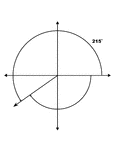### 215° and -145° Coterminal Angles

Illustration showing coterminal angles of 215° and -145°. Coterminal angles are angles drawn…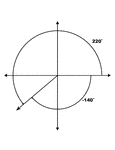### 220° and -140° Coterminal Angles

Illustration showing coterminal angles of 220° and -140°. Coterminal angles are angles drawn…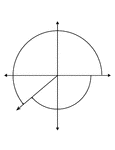### 220° and -140° Coterminal Angles

Illustration showing coterminal angles of 220° and -140°. Coterminal angles are angles drawn…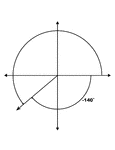### 220° and -140° Coterminal Angles

Illustration showing coterminal angles of 220° and -140°. Coterminal angles are angles drawn…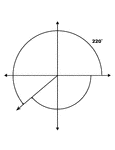### 220° and -140° Coterminal Angles

Illustration showing coterminal angles of 220° and -140°. Coterminal angles are angles drawn…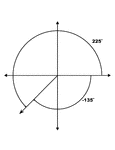### 225° and -135° Coterminal Angles

Illustration showing coterminal angles of 225° and -135°. Coterminal angles are angles drawn…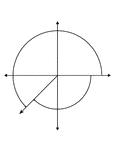### 225° and -135° Coterminal Angles

Illustration showing coterminal angles of 225° and -135°. Coterminal angles are angles drawn…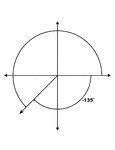### 225° and -135° Coterminal Angles

Illustration showing coterminal angles of 225° and -135°. Coterminal angles are angles drawn…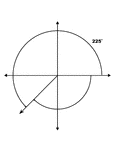### 225° and -135° Coterminal Angles

Illustration showing coterminal angles of 225° and -135°. Coterminal angles are angles drawn…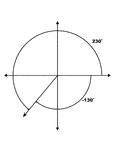### 230° and -130° Coterminal Angles

Illustration showing coterminal angles of 230° and -130°. Coterminal angles are angles drawn…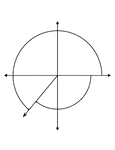### 230° and -130° Coterminal Angles

Illustration showing coterminal angles of 230° and -130°. Coterminal angles are angles drawn…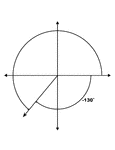### 230° and -130° Coterminal Angles

Illustration showing coterminal angles of 230° and -130°. Coterminal angles are angles drawn…### 230° and -130° Coterminal Angles

Illustration showing coterminal angles of 230° and -130°. Coterminal angles are angles drawn…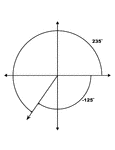### 235° and -125° Coterminal Angles

Illustration showing coterminal angles of 235° and -125°. Coterminal angles are angles drawn…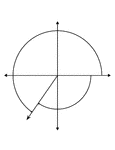### 235° and -125° Coterminal Angles

Illustration showing coterminal angles of 235° and -125°. Coterminal angles are angles drawn…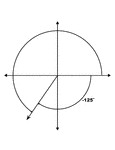### 235° and -125° Coterminal Angles

Illustration showing coterminal angles of 235° and -125°. Coterminal angles are angles drawn…### 235° and -125° Coterminal Angles

Illustration showing coterminal angles of 235° and -125°. Coterminal angles are angles drawn…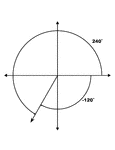### 240° and -120° Coterminal Angles

Illustration showing coterminal angles of 240° and -120°. Coterminal angles are angles drawn…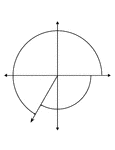### 240° and -120° Coterminal Angles

Illustration showing coterminal angles of 240° and -120°. Coterminal angles are angles drawn…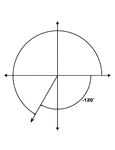### 240° and -120° Coterminal Angles

Illustration showing coterminal angles of 240° and -120°. Coterminal angles are angles drawn…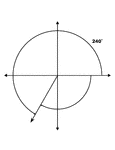### 240° and -120° Coterminal Angles

Illustration showing coterminal angles of 240° and -120°. Coterminal angles are angles drawn…### 245° and -115° Coterminal Angles

Illustration showing coterminal angles of 245° and -115°. Coterminal angles are angles drawn…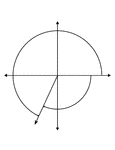### 245° and -115° Coterminal Angles

Illustration showing coterminal angles of 245° and -115°. Coterminal angles are angles drawn…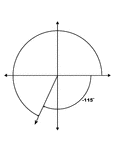### 245° and -115° Coterminal Angles

Illustration showing coterminal angles of 245° and -115°. Coterminal angles are angles drawn…### 245° and -115° Coterminal Angles

Illustration showing coterminal angles of 245° and -115°. Coterminal angles are angles drawn…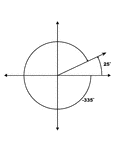### 25° and -335° Coterminal Angles

Illustration showing coterminal angles of 25° and -335°. Coterminal angles are angles drawn…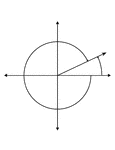### 25° and -335° Coterminal Angles

Illustration showing coterminal angles of 25° and -335°. Coterminal angles are angles drawn…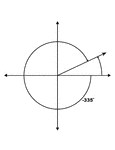### 25° and -335° Coterminal Angles

Illustration showing coterminal angles of 25° and -335°. Coterminal angles are angles drawn…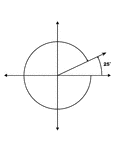### 25° and -335° Coterminal Angles

Illustration showing coterminal angles of 25° and -335°. Coterminal angles are angles drawn…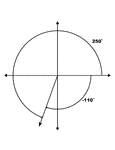### 250° and -110° Coterminal Angles

Illustration showing coterminal angles of 250° and -110°. Coterminal angles are angles drawn…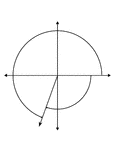### 250° and -110° Coterminal Angles

Illustration showing coterminal angles of 250° and -110°. Coterminal angles are angles drawn…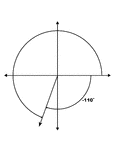### 250° and -110° Coterminal Angles

Illustration showing coterminal angles of 250° and -110°. Coterminal angles are angles drawn…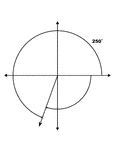### 250° and -110° Coterminal Angles

Illustration showing coterminal angles of 250° and -110°. Coterminal angles are angles drawn…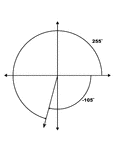### 255° and -105° Coterminal Angles

Illustration showing coterminal angles of 255° and -105°. Coterminal angles are angles drawn…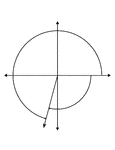### 255° and -105° Coterminal Angles

Illustration showing coterminal angles of 255° and -105°. Coterminal angles are angles drawn…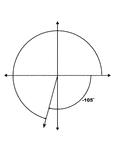### 255° and -105° Coterminal Angles

Illustration showing coterminal angles of 255° and -105°. Coterminal angles are angles drawn…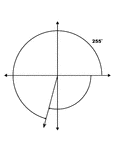### 255° and -105° Coterminal Angles

Illustration showing coterminal angles of 255° and -105°. Coterminal angles are angles drawn…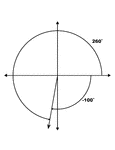### 260° and -100° Coterminal Angles

Illustration showing coterminal angles of 260° and -100°. Coterminal angles are angles drawn…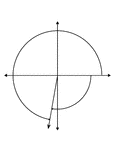### 260° and -100° Coterminal Angles

Illustration showing coterminal angles of 260° and -100°. Coterminal angles are angles drawn…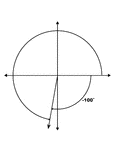### 260° and -100° Coterminal Angles

Illustration showing coterminal angles of 260° and -100°. Coterminal angles are angles drawn…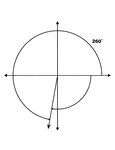### 260° and -100° Coterminal Angles

Illustration showing coterminal angles of 260° and -100°. Coterminal angles are angles drawn…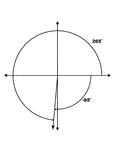### 265° and -95° Coterminal Angles

Illustration showing coterminal angles of 265° and -95°. Coterminal angles are angles drawn…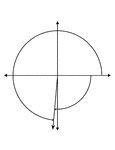### 265° and -95° Coterminal Angles

Illustration showing coterminal angles of 265° and -95°. Coterminal angles are angles drawn…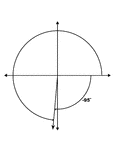### 265° and -95° Coterminal Angles

Illustration showing coterminal angles of 265° and -95°. Coterminal angles are angles drawn…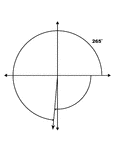### 265° and -95° Coterminal Angles

Illustration showing coterminal angles of 265° and -95°. Coterminal angles are angles drawn…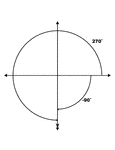### 270° and -90° Coterminal Angles

Illustration showing coterminal angles of 270° and -90°. Coterminal angles are angles drawn…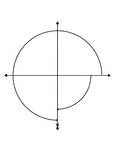### 270° and -90° Coterminal Angles

Illustration showing coterminal angles of 270° and -90°. Coterminal angles are angles drawn…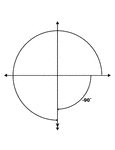### 270° and -90° Coterminal Angles

Illustration showing coterminal angles of 270° and -90°. Coterminal angles are angles drawn…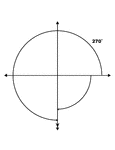### 270° and -90° Coterminal Angles

Illustration showing coterminal angles of 270° and -90°. Coterminal angles are angles drawn…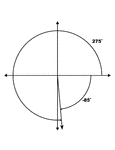### 275° and -85° Coterminal Angles

Illustration showing coterminal angles of 275° and -85°. Coterminal angles are angles drawn…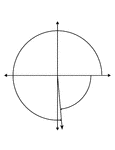### 275° and -85° Coterminal Angles

Illustration showing coterminal angles of 275° and -85°. Coterminal angles are angles drawn…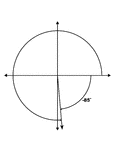### 275° and -85° Coterminal Angles

Illustration showing coterminal angles of 275° and -85°. Coterminal angles are angles drawn…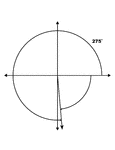### 275° and -85° Coterminal Angles

Illustration showing coterminal angles of 275° and -85°. Coterminal angles are angles drawn…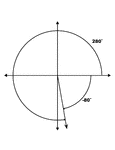### 280° and -80° Coterminal Angles

Illustration showing coterminal angles of 280° and -80°. Coterminal angles are angles drawn…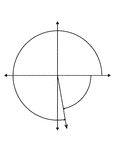### 280° and -80° Coterminal Angles

Illustration showing coterminal angles of 280° and -80°. Coterminal angles are angles drawn…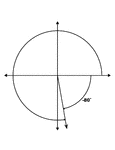### 280° and -80° Coterminal Angles

Illustration showing coterminal angles of 280° and -80°. Coterminal angles are angles drawn…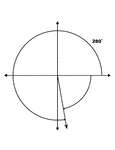### 280° and -80° Coterminal Angles

Illustration showing coterminal angles of 280° and -80°. Coterminal angles are angles drawn…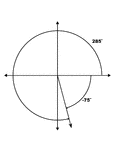### 285° and -75° Coterminal Angles

Illustration showing coterminal angles of 285° and -75°. Coterminal angles are angles drawn…### 285° and -75° Coterminal Angles

Illustration showing coterminal angles of 285° and -75°. Coterminal angles are angles drawn…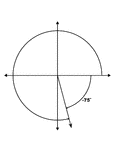### 285° and -75° Coterminal Angles

Illustration showing coterminal angles of 285° and -75°. Coterminal angles are angles drawn…